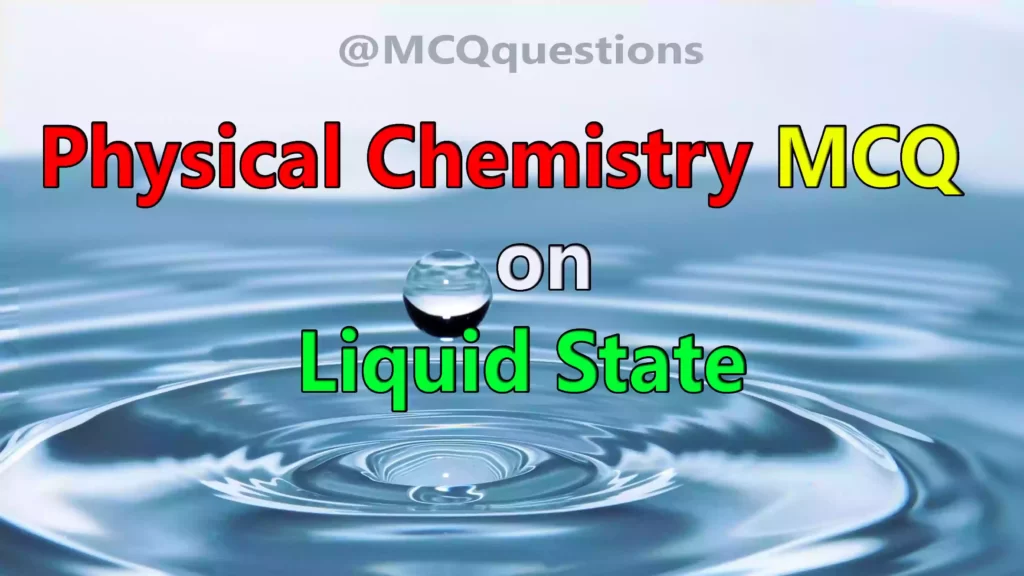# Physical Chemistry MCQ on Liquid State

## Physical Chemistry MCQ on Liquid state

1. The boiling point at pressure 1 atm is called

(a) Standard boiling point

(b) Normal boiling point

(c) Van der Waal boiling point

(d) Saturated boiling point

`Answer: (c) Van der Waal boiling point`

2. Viscosity of liquid _________ with rise in temperature.

(a) Increases

(b) Decreases

(c) Remains constant

(d) Is independent

3. Path in liquid in which layers do not meet each other is _______

(a) laminar flow

(b) tubular flow

(c) viscosity

(d) straight path

4.What is S.I. unit of Surface Tension?

(a) Dyne/meter

(b) Newton-meter

(c) Newton/meter

(d) Dyne-meter

5. A water drop is spherical in shape due to ____________

(a) Viscosity

(b) Poise

(c) Surface tension

(d) Reflection

6.At critical temperature,the surface tension will be

(a) one

(b) zero

(c) two

(d) three

7. Higher the viscosity, the slower the liquid flows.

(a) True

(b) False

8. : The vapor pressure is directly proportional to the temperature because of

(a) more kinetic energy only

(b) faster particle movement only

(c) more potential energy

(d) more kinetic energy and faster particle movement

`Answer (d) more kinetic energy and faster particle movement`

9. When the movement of water molecules in liquid becomes equal to the molecules in vapor form, a state is called

(a) equilibrium

(b) constant

(c) ideal

(d) non-ideal

10.The state of freezing involves

(a) loss of kinetic energy

(b) increased force of attraction

(c) liquid solidifies

(d) all of above